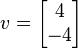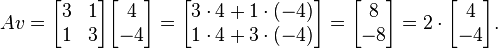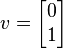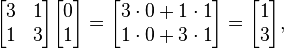## Eigenvectors and Eigenvalues Calculation

Regular Matrix A = Identity Matrix I =
Scalar Matrix(Z=c×I) c =
|A| =
Singular Matrix (A - c×I) =
|A - c×I| =
Trace of A =
Eigen Value (c2) =
Eigen Value (c1) =
c1 in Eigen Vector x1 value =
c2 in Eigen Vector x1 value =
c1 in Eigen Vector x2 value =
c2 in Eigen Vector x2 value =

An eigenvector of a square matrix A is a non-zero vector v, When A v = λ v, the λ is called the eigenvalue of A corresponding to v.

All eigenvalues and eigenvectors satisfy the equation Ax = λx for a given square matrix A.

The online Eigenvectors and Eigenvalues Calculator can get |A|, Singular Matrix (A - c×I), Trace of A, Eigen Value of the matrix A

### For example

For matrixthe vectoris an eigenvector with eigenvalue 2.On the other hand the vectoris not an eigenvector, sinceand this vector is not a multiple of the original vector v.

Thinkcalculator.com provides you helpful and handy calculator resources.